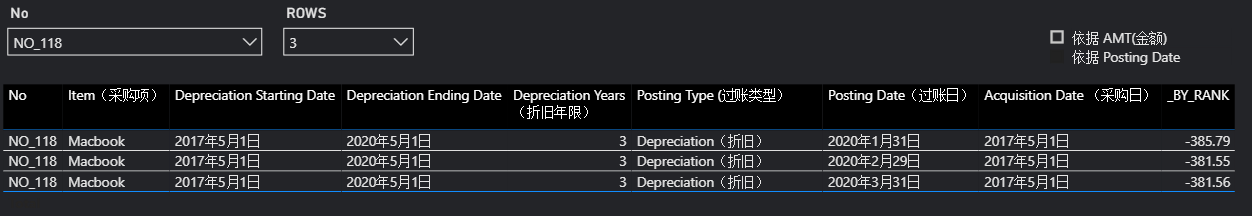# DAX应用：控制表格显示行数

（本文要解决的问题：利用DAX度量值，控制前端表格显示行数）

### 需求描述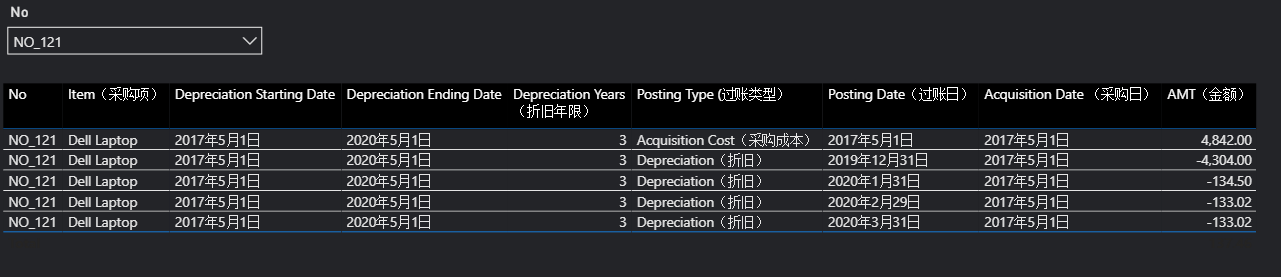### 解决思路

``````MAX_INDEX =
VAR MAX_1 = CALCULATE(MAX('Dataset'[Index]),
FILTER(ALLEXCEPT('Dataset','Dataset'[No]),
NOT ISBLANK(SUM('Dataset'[Amount（发生额）]))))
RETURN
MAX_1
``````

``````PARA_SHOWLASTENTRY = DATATABLE("NAME",STRING,Show last only)
``````

``````AMT_ONE =
IF(ISFILTERED(PARA_SHOWLASTENTRY[NAME]),
IF(MAX('Dataset'[index]) = [MAX_INDEX],[AMT],BLANK())
,[AMT])
``````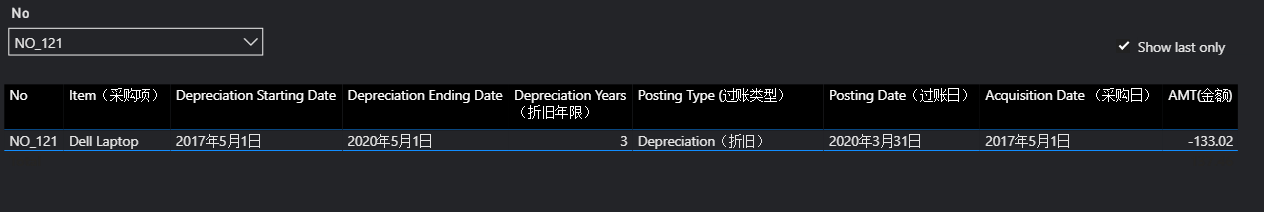### 最终方案

``````PARA_BY =
DATATABLE("NAME",STRING,"VALUE",INTEGER,
{
{"依据 Posting Date",0},
{"依据 AMT(金额)",1}
})
``````

``````_RANK =
SWITCH (
SELECTEDVALUE ( PARA_BY[VALUE] ),
0, RANKX (
ALLSELECTED ( 'Dataset' ),
FIRSTNONBLANK ( 'Dataset'[Posting Date（过账日）], 0 ),
,
DESC,
SKIP
),
1, RANKX ( ALLSELECTED ( 'Dataset' ), [AMT],, DESC, SKIP )
)

``````

``````_BY_RANK = IF([_RANK]=1,[AMT],BLANK())
``````

• 默认状态：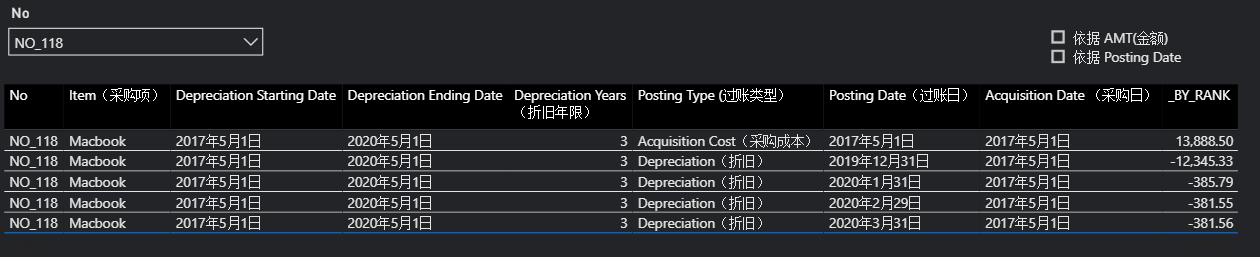• 选择”依据AMT(金额)”: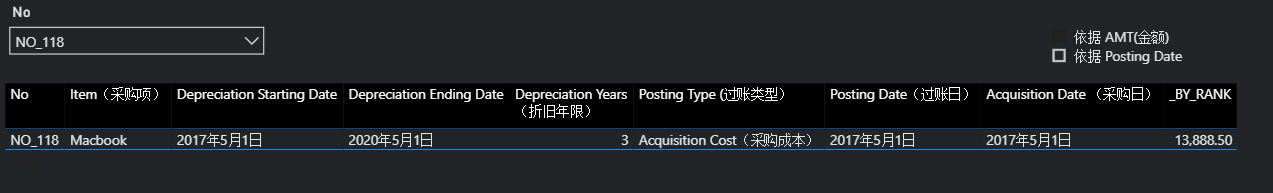• 选择”依据Posting Date”: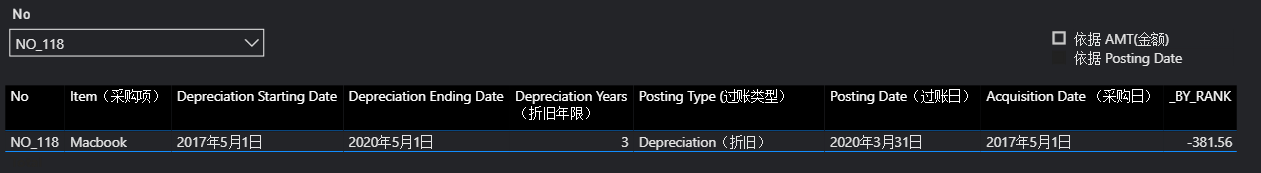更进一步地，也可以将_BY_RANK度量值中的1设为变量(what-if参数)，交由用户设定：

``````_BY_RANK = IF([_RANK]<='ROWS'[ROWS Value],[AMT],BLANK())
``````